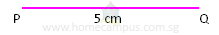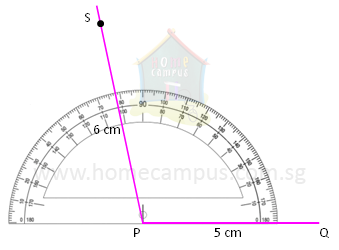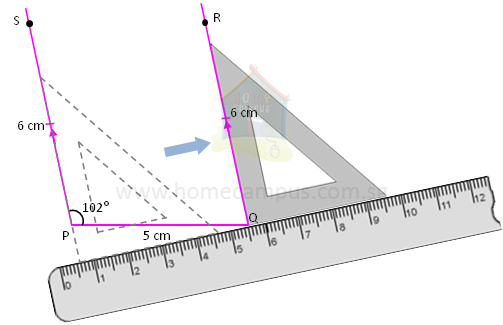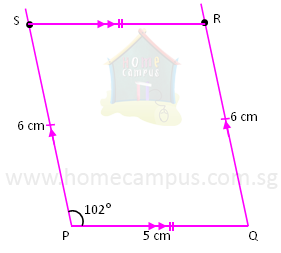## How to Draw a Parallelogram?

Practice Unlimited Questions

#### 1. Draw a parallelogram PQRS so that ∠SPQ = 110°, PQ = 5 cm and PS = 6 cm.

Step 1: Draw a straight line PQ 5 cm long.Step 2: Using a protractor draw an angle of 102° from Point P. Mark the Point S so that PS = 6 cm.Step 3: Using a ruler and a set square draw a line QR so that it is parallel to PS. Mark the Point R so that QR = 6 cm.Step 4: Join Point S to Point R.PQRS is the required parallelogram.